# Test Prep Plan - Take a practice test

Take this practice test to check your existing knowledge of the course material. We'll review your answers and create a Test Prep Plan for you based on your results.
How Test Prep Plans work
1
2Based on your results, we'll create a customized Test Prep Plan just for you!
3Study smarter
Study more effectively: skip concepts you already know and focus on what you still need to learn.

# Circular Arc & Circles in Coordinate Geometry Chapter Exam

Exam Instructions:

Choose your answers to the questions and click 'Next' to see the next set of questions. You can skip questions if you would like and come back to them later with the yellow "Go To First Skipped Question" button. When you have completed the practice exam, a green submit button will appear. Click it to see your results. Good luck!

### Page 1

#### Question 1 1. What is the area of the shaded area, rounded to the nearest whole number?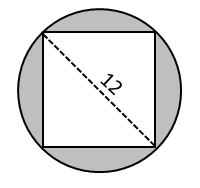#### Question 3 3. In the picture below, if major arc BC is 258 degrees and minor arc BC is 102 degrees, what is the measure of angle BAC?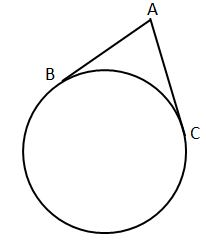#### Question 4 4. In the picture below, if arc AB is 82 degrees, what is the measure of angle BAC?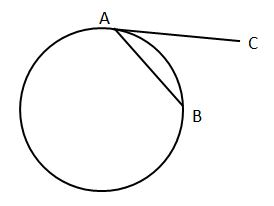#### Question 5 5. In the picture below, if AE is 8, CE is 12 and ED is 5, what is the length of EB?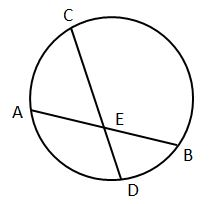### Page 2

#### Question 6 6. In the picture below, if AB is 12, AC is 24 and AD is 10, what is the length of AE?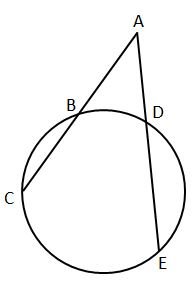#### Question 9 9. Chord AB measures 6. Chord GI measures 6. Line segment CD measures 3. What does line segment CF measure?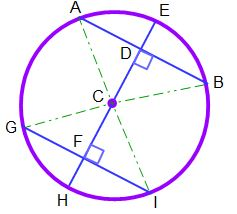#### Question 10 10. Chord AB measures 12. Radius CE measures 8. What does line segment AD measure?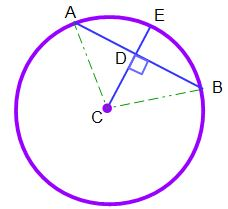### Page 3

#### Question 13 13. Which line is a tangent to the pictured circle?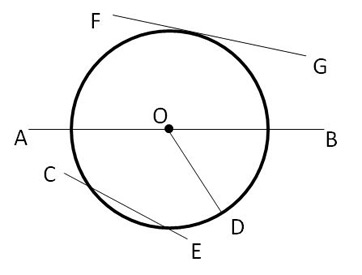### Page 4

#### Question 16 16. Find the center and radius of the following equation: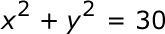#### Question 17 17. If the measure of central angle AOB is 62 degrees, what is the measure of inscribed angle ACB?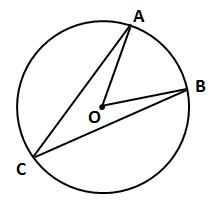#### Question 18 18. If arc BC is 84 degrees, what is the measure of angle ACB?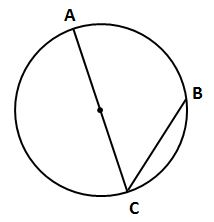#### Question 19 19. If angle AOB is 40 degrees, what is the measure of angle ACB?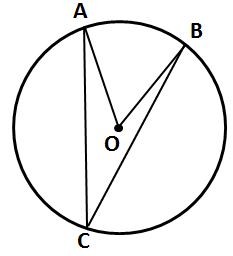#### Question 20 20. If angle ACB is 34 degrees, what is the measure of angle ADB?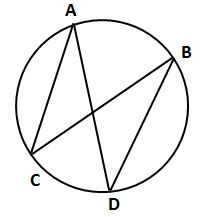### Page 6

#### Question 26 26. In the picture below, if arc BD is 168 degrees and arc BC is 70 degrees, what is the measure of angle BAD?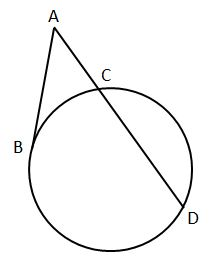#### Question 27 27. In the picture below, if AC is 14 and AB is 10, what is the length of AD?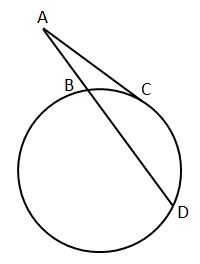#### Circular Arc & Circles in Coordinate Geometry Chapter Exam Instructions

Choose your answers to the questions and click 'Next' to see the next set of questions. You can skip questions if you would like and come back to them later with the yellow "Go To First Skipped Question" button. When you have completed the practice exam, a green submit button will appear. Click it to see your results. Good luck!

Support